# Collectibles And Antiques

### Auction Details

Collectibles And Antiques
Choose Your Layout >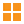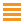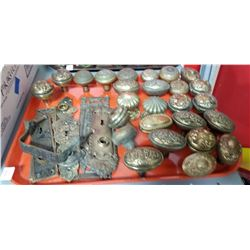1 - Shelf One
CDC Auctions
Shelf One
Bidding Has Concluded

Sold to n*l for (10.00 + 1.30) x 1 = 11.30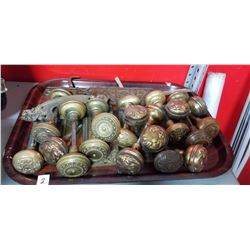2 - Shelf One
CDC Auctions
Shelf One
Bidding Has Concluded

Sold to f***r for (12.50 + 1.63) x 1 = 14.13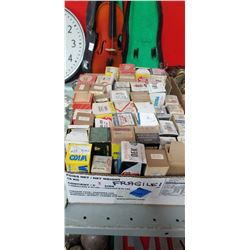3 - Shelf One
CDC Auctions
Shelf One
Bidding Has Concluded

Sold to f***r for (22.50 + 2.93) x 1 = 25.43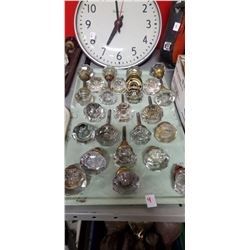4 - Shelf One
CDC Auctions
Shelf One
Bidding Has Concluded

Sold to f***r for (22.50 + 2.93) x 1 = 25.43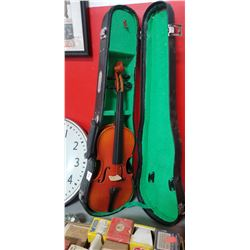5 - Shelf One
CDC Auctions
Shelf One
Bidding Has Concluded

Sold to f***r for (15.00 + 1.95) x 1 = 16.95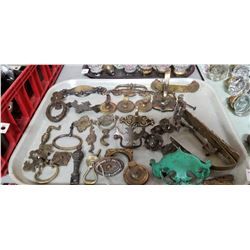7 - Shelf One
CDC Auctions
Shelf One
Bidding Has Concluded

Sold to f***r for (12.50 + 1.63) x 1 = 14.13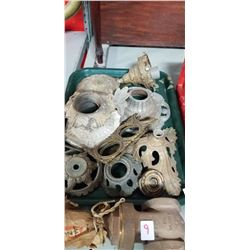10 - Shelf One
CDC Auctions
Shelf One
Bidding Has Concluded

Sold to f***r for (5.00 + 0.65) x 1 = 5.6511 - Shelf One
CDC Auctions
Shelf One
Bidding Has Concluded

Sold to f***r for (25.00 + 3.25) x 1 = 28.25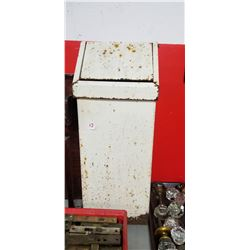12 - Shelf One
CDC Auctions
Shelf One
Bidding Has Concluded

Sold to f***r for (5.00 + 0.65) x 1 = 5.65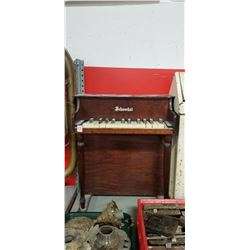13 - Shelf One
CDC Auctions
Shelf One
Bidding Has Concluded

Sold to f***r for (15.00 + 1.95) x 1 = 16.95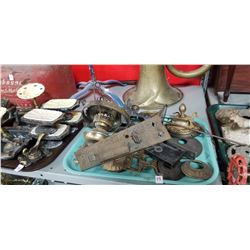14 - Shelf One
CDC Auctions
Shelf One
Bidding Has Concluded

Sold to f***r for (15.00 + 1.95) x 1 = 16.95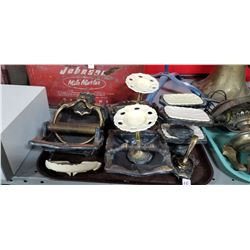15 - Shelf One
CDC Auctions
Shelf One
Bidding Has Concluded

Sold to f***r for (22.50 + 2.93) x 1 = 25.43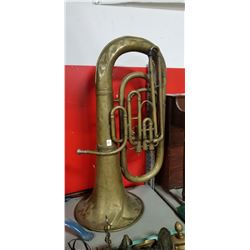16 - Shelf Two
CDC Auctions
Shelf Two
Bidding Has Concluded

Sold to f***r for (22.50 + 2.93) x 1 = 25.43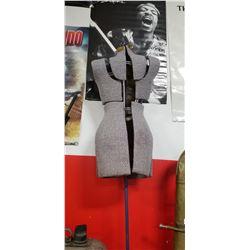17 - Shelf One
CDC Auctions
Shelf One
Bidding Has Concluded

Sold to f***r for (17.50 + 2.28) x 1 = 19.78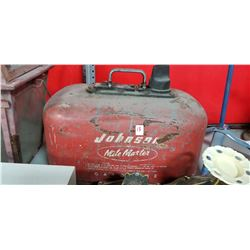18 - Shelf One
CDC Auctions
Shelf One
Bidding Has Concluded

Sold to f***r for (7.50 + 0.98) x 1 = 8.48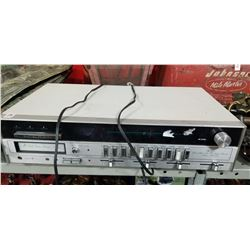19 - Shelf One
CDC Auctions
Shelf One
Bidding Has Concluded

Sold to d*****S for (10.00 + 1.30) x 1 = 11.30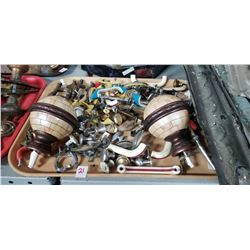21 - Shelf One
CDC Auctions
Shelf One
Bidding Has Concluded

Sold to f***r for (5.00 + 0.65) x 1 = 5.65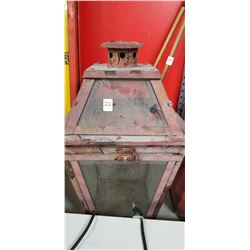22 - Shelf One
CDC Auctions
Shelf One
Bidding Has Concluded

Sold to f***r for (15.00 + 1.95) x 1 = 16.95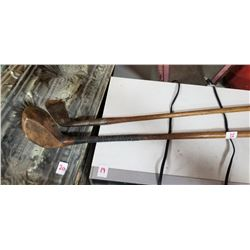23 - Shelf One
CDC Auctions
Shelf One
Bidding Has Concluded

Sold to f***r for (22.50 + 2.93) x 1 = 25.43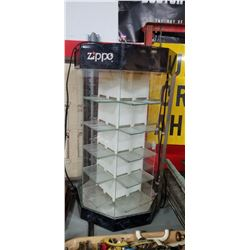24 - Shelf One
CDC Auctions
Shelf One
Bidding Has Concluded

Sold to f***r for (35.00 + 4.55) x 1 = 39.5525 - Shelf One
CDC Auctions
Shelf One
Bidding Has Concluded

Sold to f***r for (85.00 + 11.05) x 1 = 96.05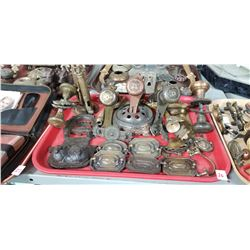26 - Shelf One
CDC Auctions
Shelf One
Bidding Has Concluded

Sold to f***r for (22.50 + 2.93) x 1 = 25.43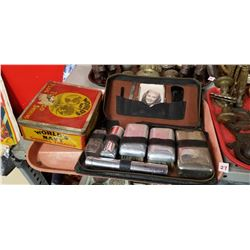27 - Shelf One
CDC Auctions
Shelf One
Bidding Has Concluded

Sold to f***r for (5.00 + 0.65) x 1 = 5.65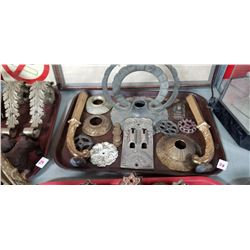28 - Shelf One
CDC Auctions
Shelf One
Bidding Has Concluded

Sold to f***r for (45.00 + 5.85) x 1 = 50.85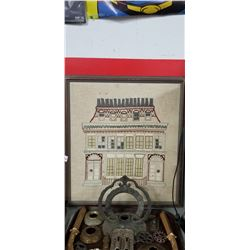30 - Shelf One
CDC Auctions
Shelf One
Bidding Has Concluded

Sold to f***r for (7.50 + 0.98) x 1 = 8.48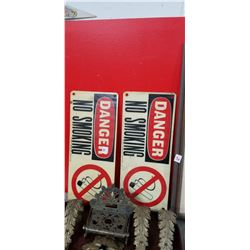31 - Shelf One
CDC Auctions
Shelf One
Bidding Has Concluded

Sold to f***r for (22.50 + 2.93) x 1 = 25.43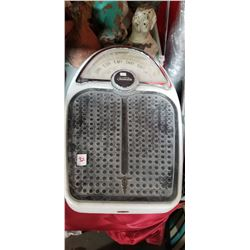32 - Shelf One
CDC Auctions
Shelf One
Bidding Has Concluded

Sold to f***r for (5.00 + 0.65) x 1 = 5.65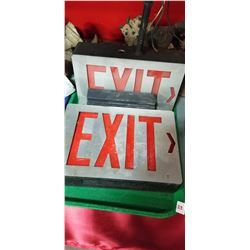33 - Shelf One
CDC Auctions
Shelf One
Bidding Has Concluded

Sold to f***r for (25.00 + 3.25) x 1 = 28.25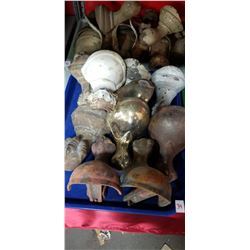34 - Shelf Two
CDC Auctions
Shelf Two
Bidding Has Concluded

Sold to f***r for (80.00 + 10.40) x 1 = 90.4035 - Shelf One
CDC Auctions
Shelf One
Bidding Has Concluded

Sold to f***r for (50.00 + 6.50) x 1 = 56.50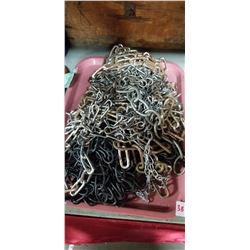38 - Shelf One
CDC Auctions
Shelf One
Bidding Has Concluded

Sold to f***r for (15.00 + 1.95) x 1 = 16.95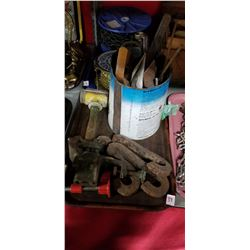39 - Shelf One
CDC Auctions
Shelf One
Bidding Has Concluded

Sold to f***r for (5.00 + 0.65) x 1 = 5.65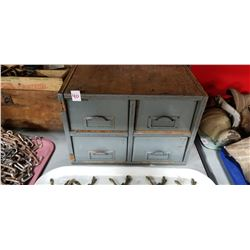40 - Shelf One
CDC Auctions
Shelf One
Bidding Has Concluded

Sold to f***r for (5.00 + 0.65) x 1 = 5.65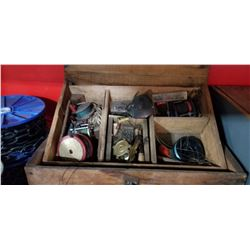41 - Shelf One
CDC Auctions
Shelf One
Bidding Has Concluded

Sold to f***r for (25.00 + 3.25) x 1 = 28.25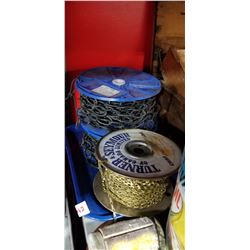42 - Shelf One
CDC Auctions
Shelf One
Bidding Has Concluded

Sold to f***r for (5.00 + 0.65) x 1 = 5.65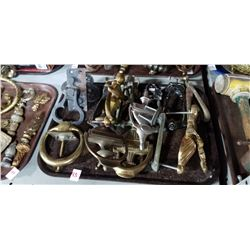43 - Shelf One
CDC Auctions
Shelf One
Bidding Has Concluded

Sold to f***r for (20.00 + 2.60) x 1 = 22.60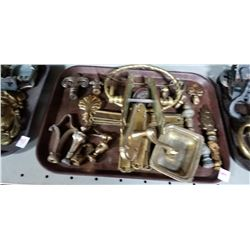44 - Shelf One
CDC Auctions
Shelf One
Bidding Has Concluded

Sold to f***r for (10.00 + 1.30) x 1 = 11.30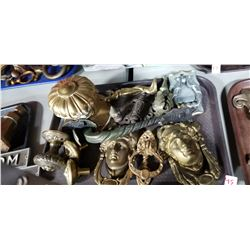45 - Shelf One
CDC Auctions
Shelf One
Bidding Has Concluded

Sold to f***r for (35.00 + 4.55) x 1 = 39.55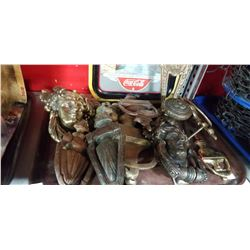46 - Shelf One
CDC Auctions
Shelf One
Bidding Has Concluded

Sold to f***r for (60.00 + 7.80) x 1 = 67.80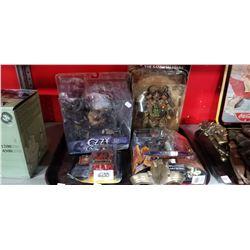47 - Shelf One
CDC Auctions
Shelf One
Bidding Has Concluded

Sold to f***r for (35.00 + 4.55) x 1 = 39.55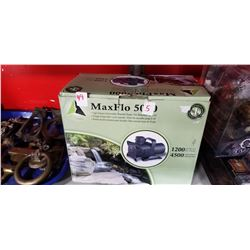49 - Shelf One
CDC Auctions
Shelf One
Bidding Has Concluded

Sold to M*******h for (10.00 + 1.30) x 1 = 11.30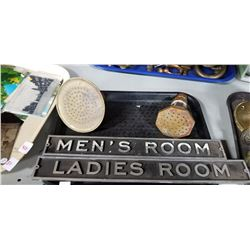50 - Shelf One
CDC Auctions
Shelf One
Bidding Has Concluded

Sold to f***r for (15.00 + 1.95) x 1 = 16.95